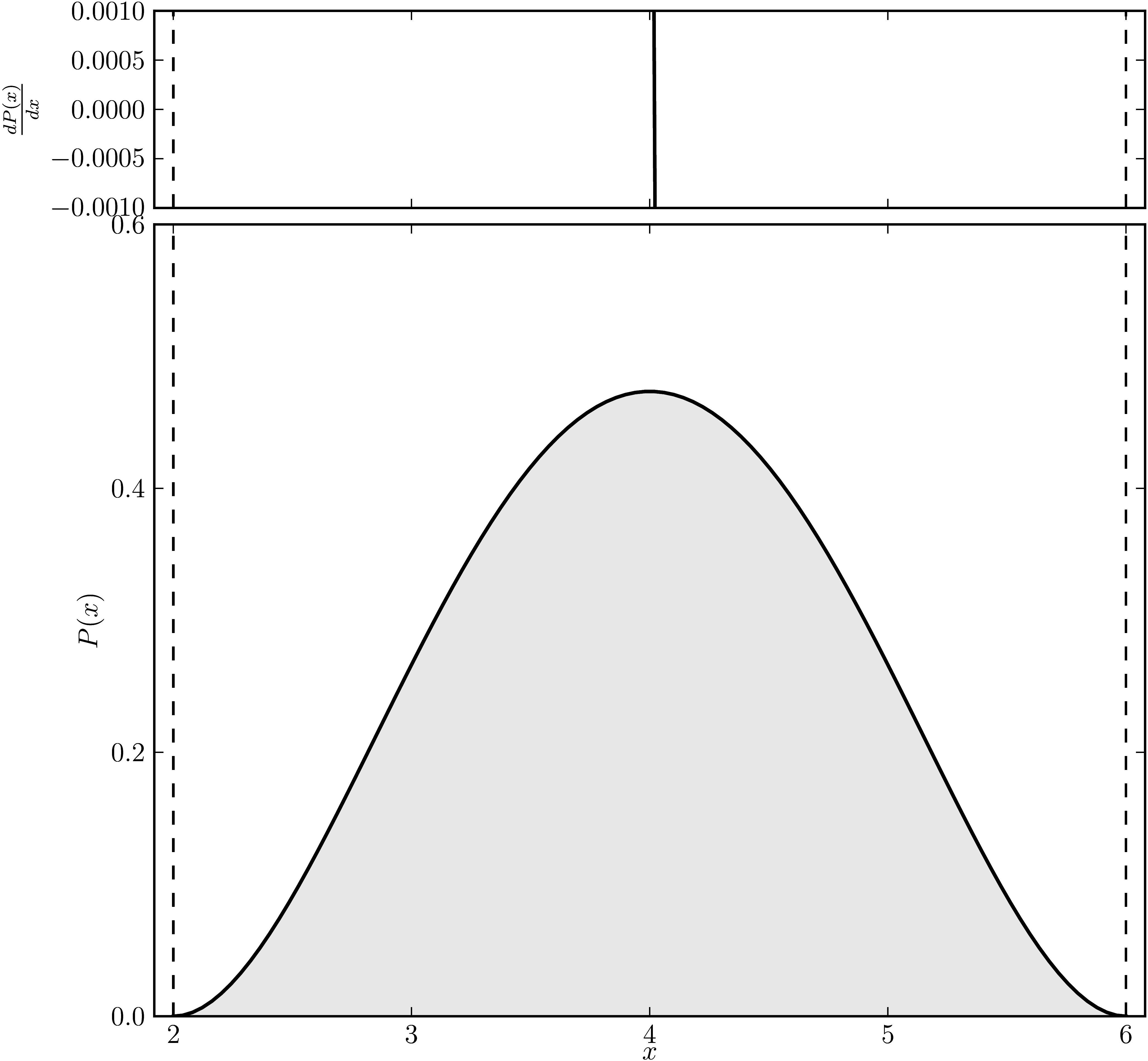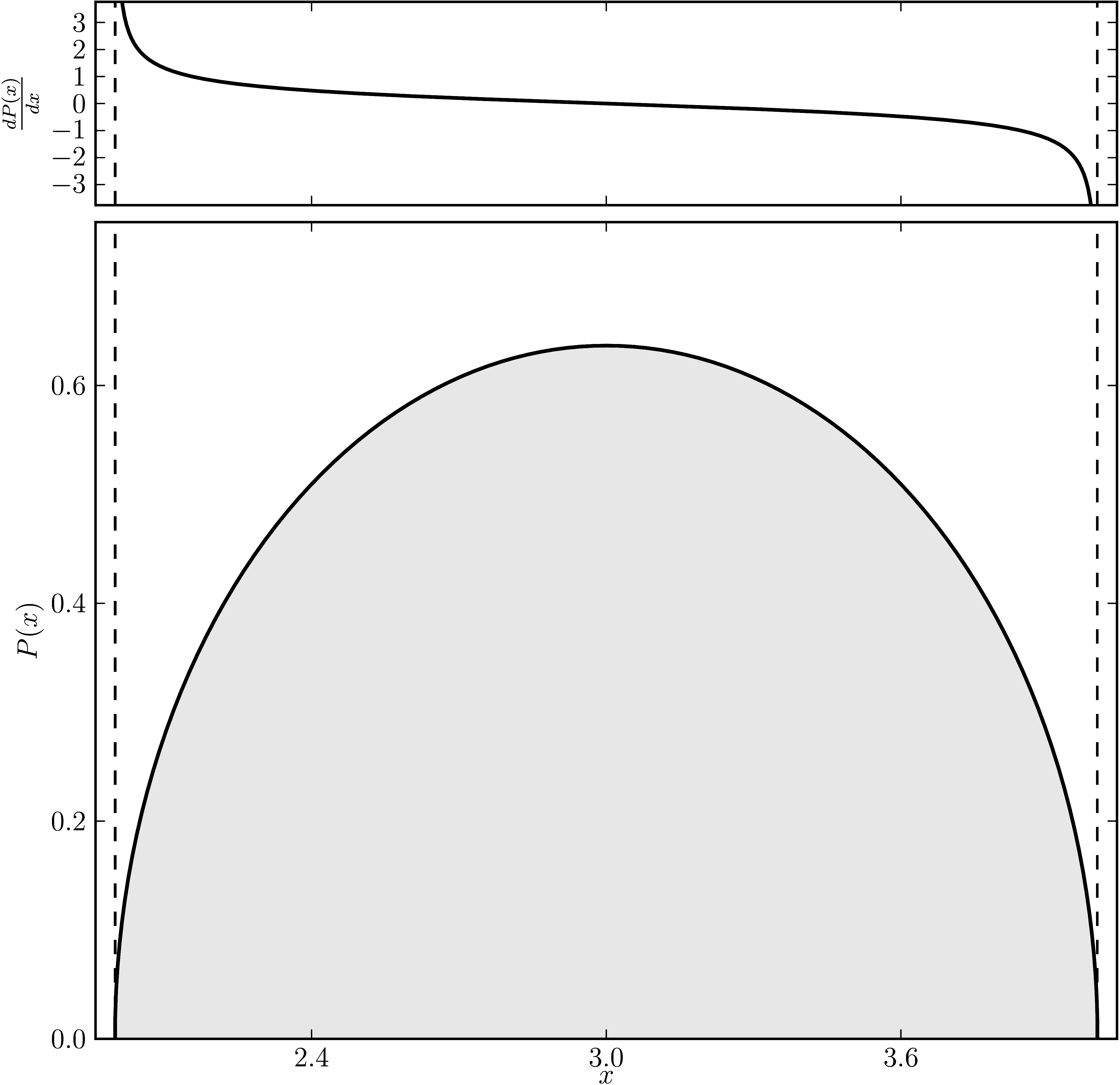# Hermitian matrices

In the case of Hermitian matrices the numerical shadow is a one dimensional distribution.

## Diagonal matrices

### Example 1

The matrix is $\mathrm{diag}(1, 2, 3, 4 ,5, 6)$. The dashed lines mark the eigenvalues. The upped plot shows the derivative of the distribution.

Standard numerical shadow with respect to complex states Numerical shadow with respect to real states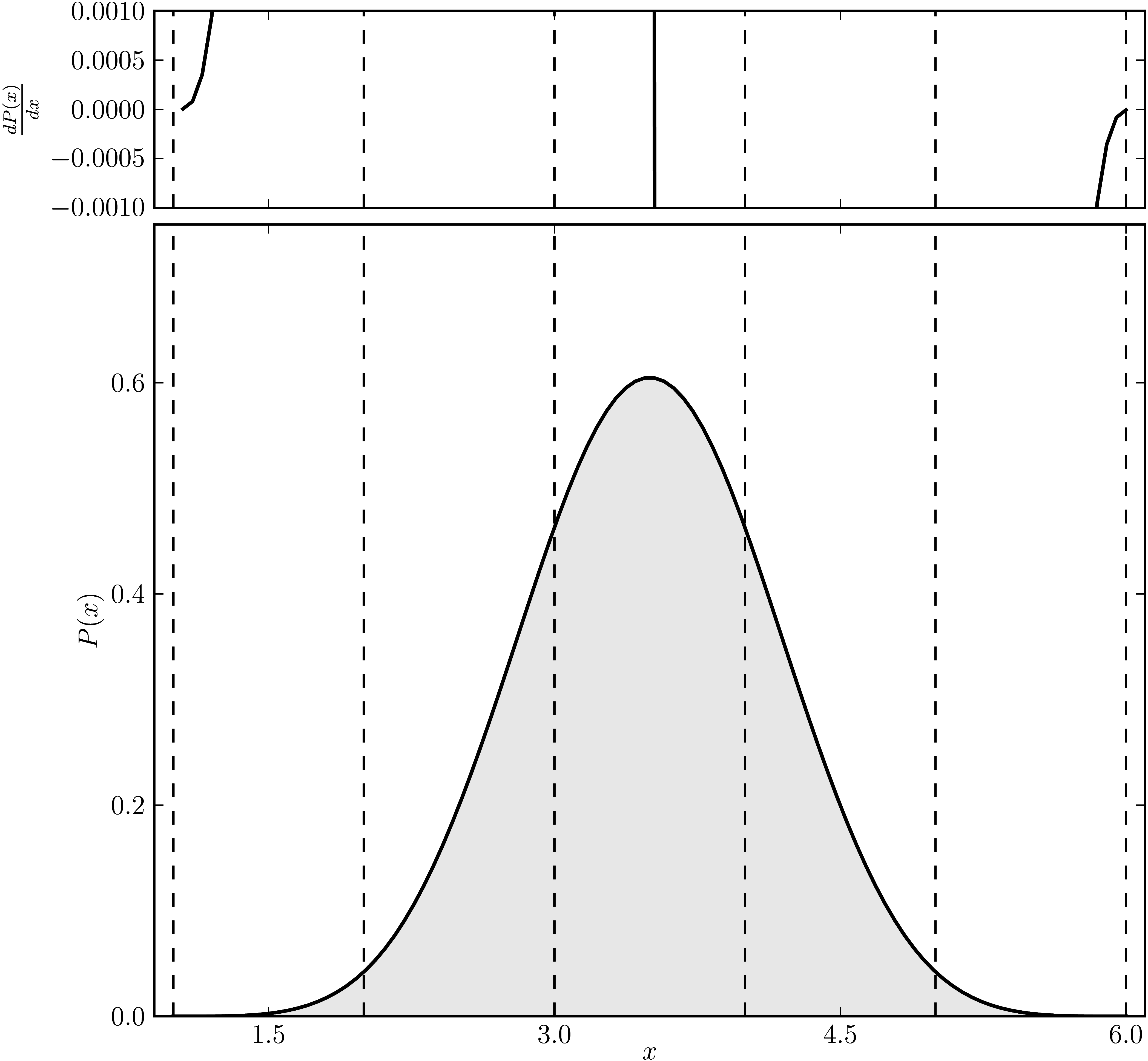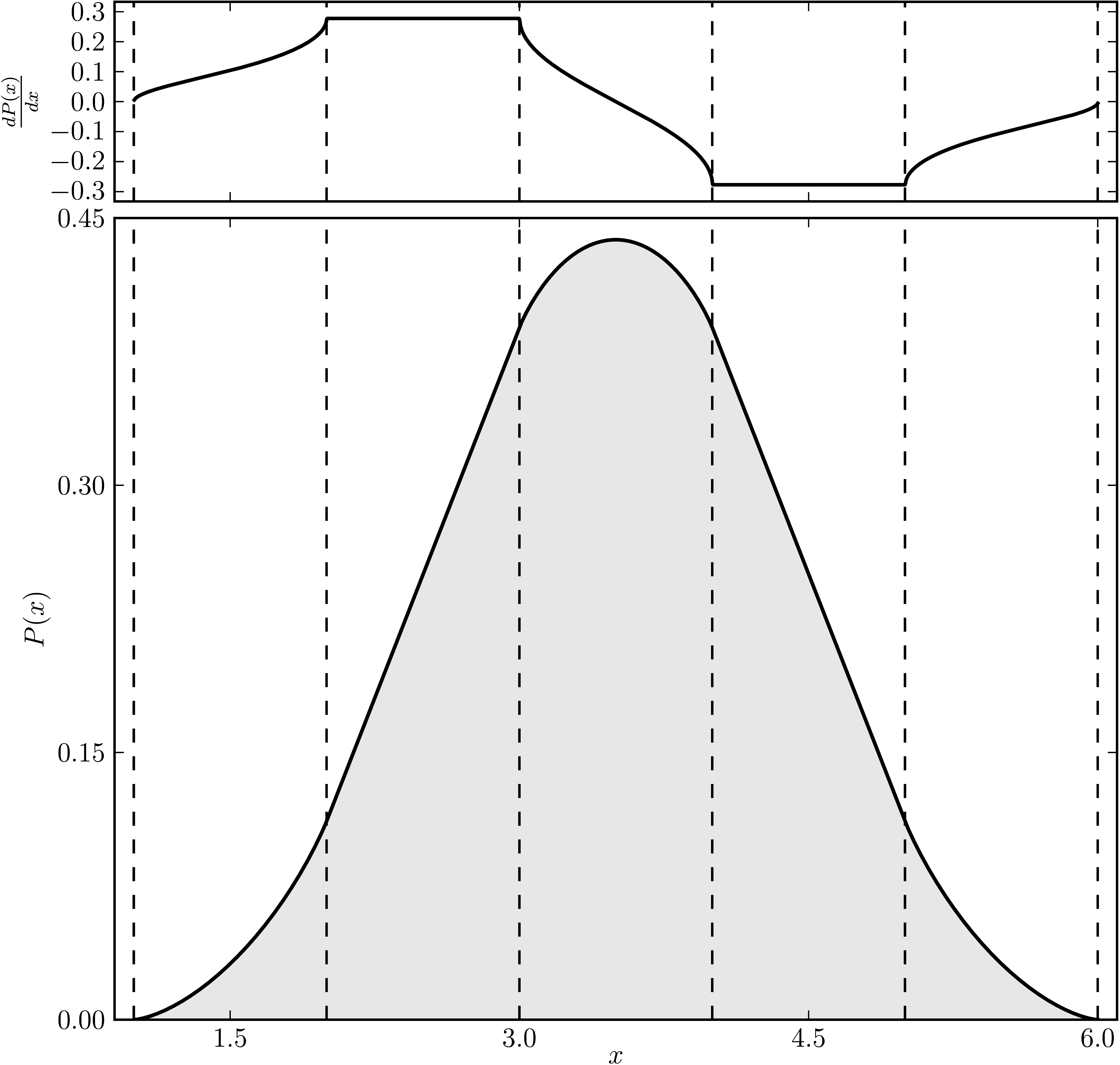### Example 2

The matrix is $\mathrm{diag}(1, 2, 2, 4 ,5, 6)$. The dashed lines mark the eigenvalues. The upped plot shows the derivative of the distribution.

Standard numerical shadow with respect to complex states Numerical shadow with respect to real states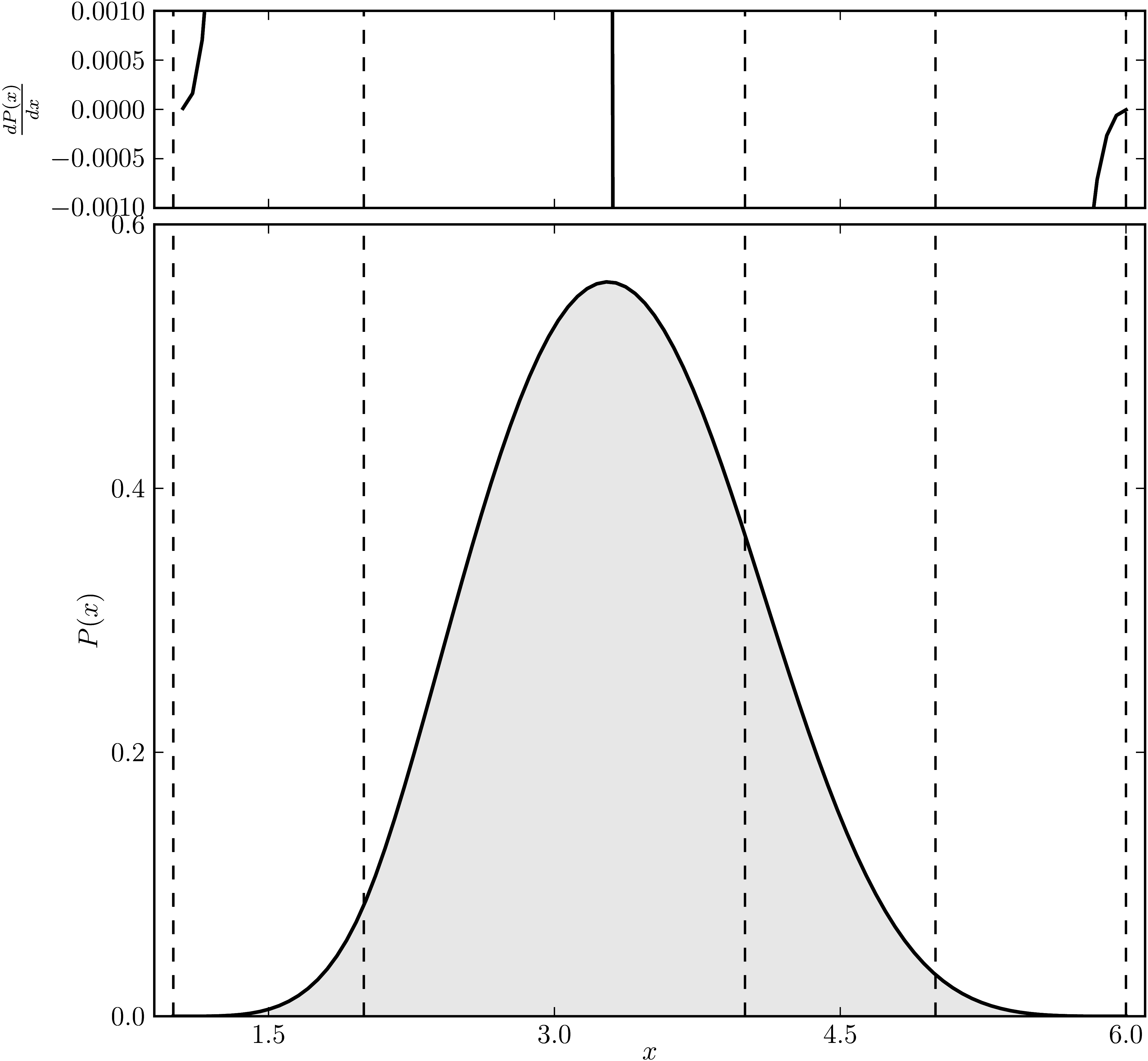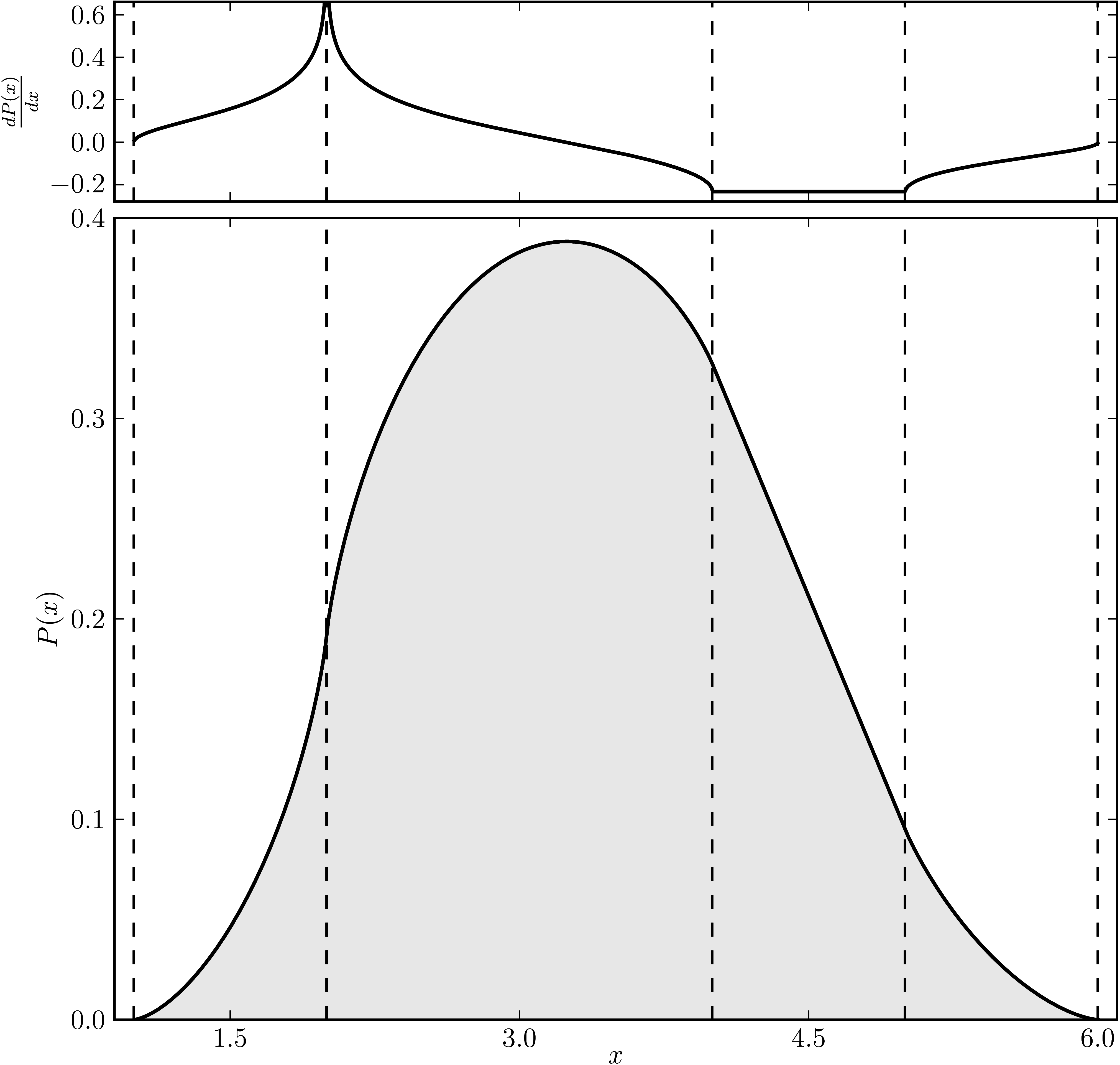### Example 3

The matrix is $\mathrm{diag}(1, 2, 2, 4 ,4, 6)$. The dashed lines mark the eigenvalues. The upped plot shows the derivative of the distribution.

Standard numerical shadow with respect to complex states Numerical shadow with respect to real states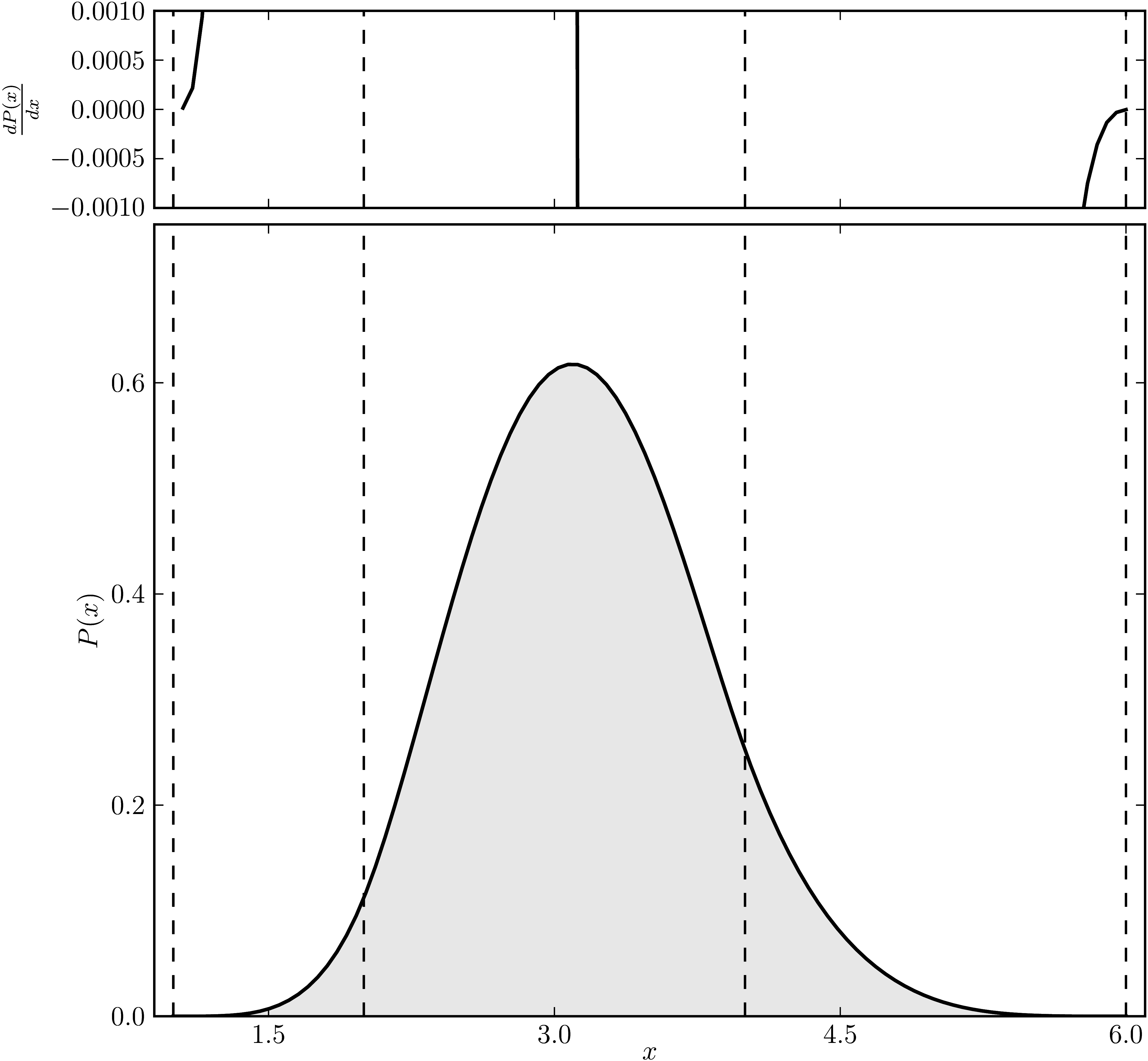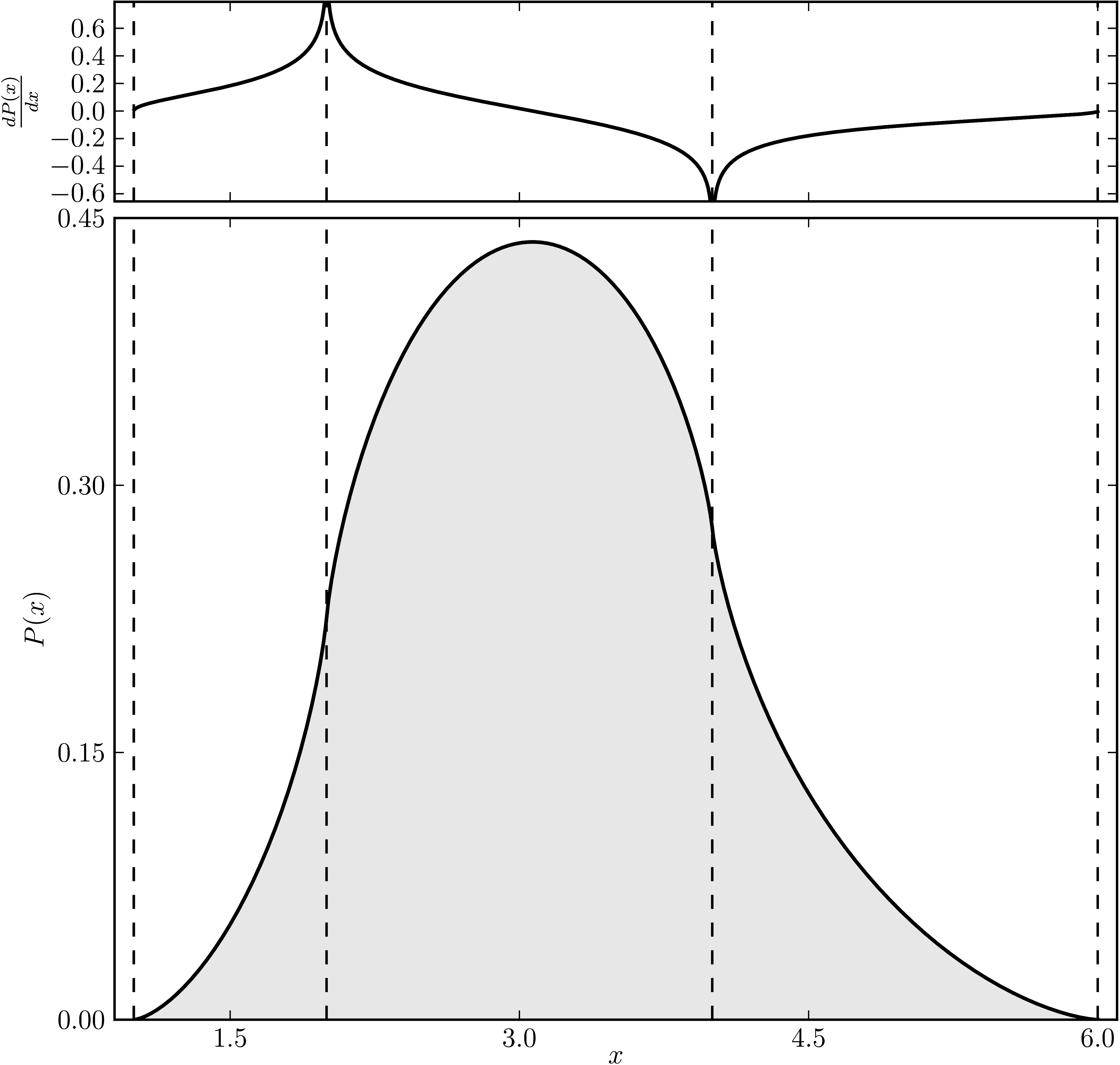### Example 4

The matrix is $\mathrm{diag}(1, 2, 2, 2 ,5, 6)$. The dashed lines mark the eigenvalues. The upped plot shows the derivative of the distribution.

Standard numerical shadow with respect to complex states Numerical shadow with respect to real states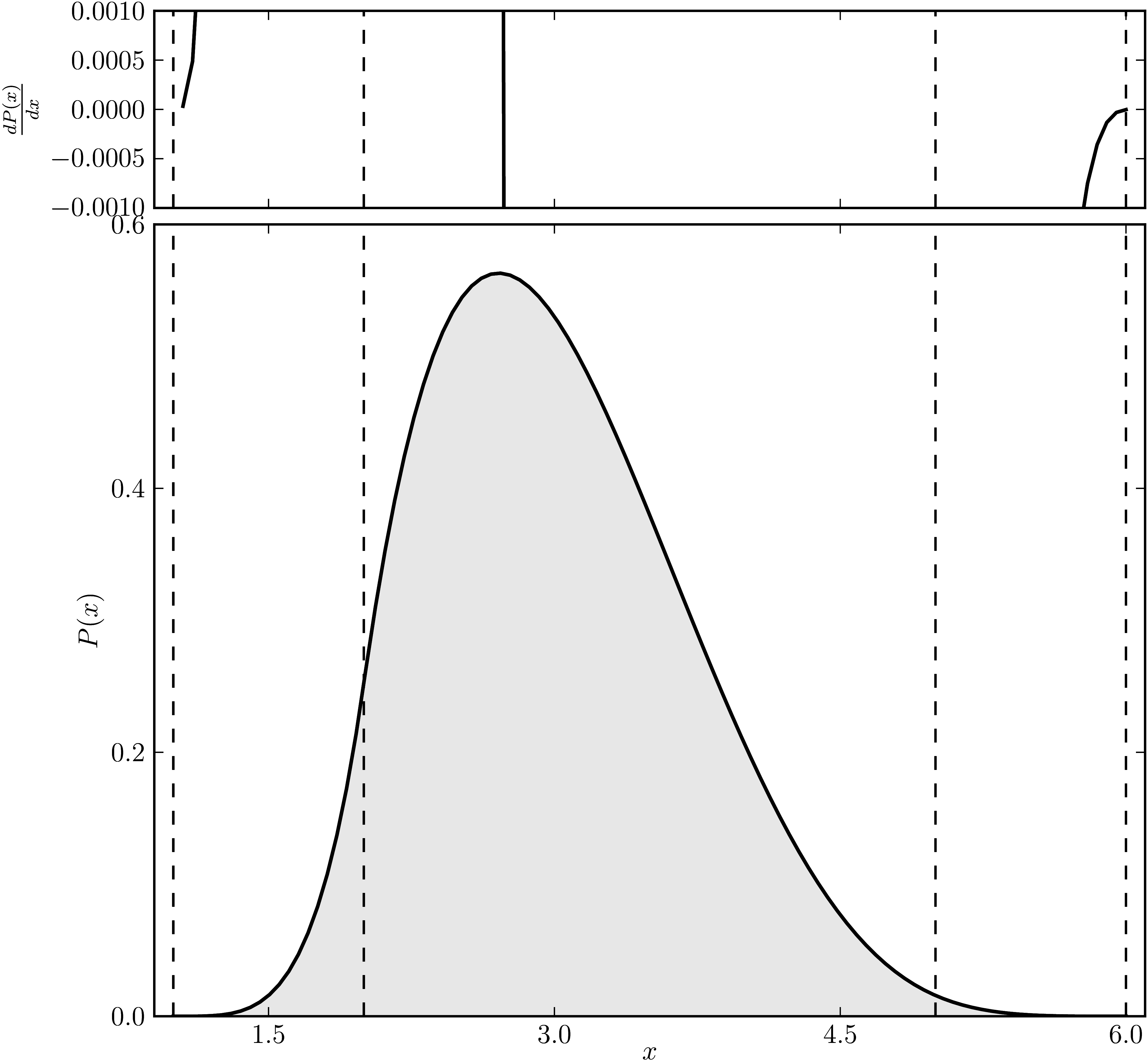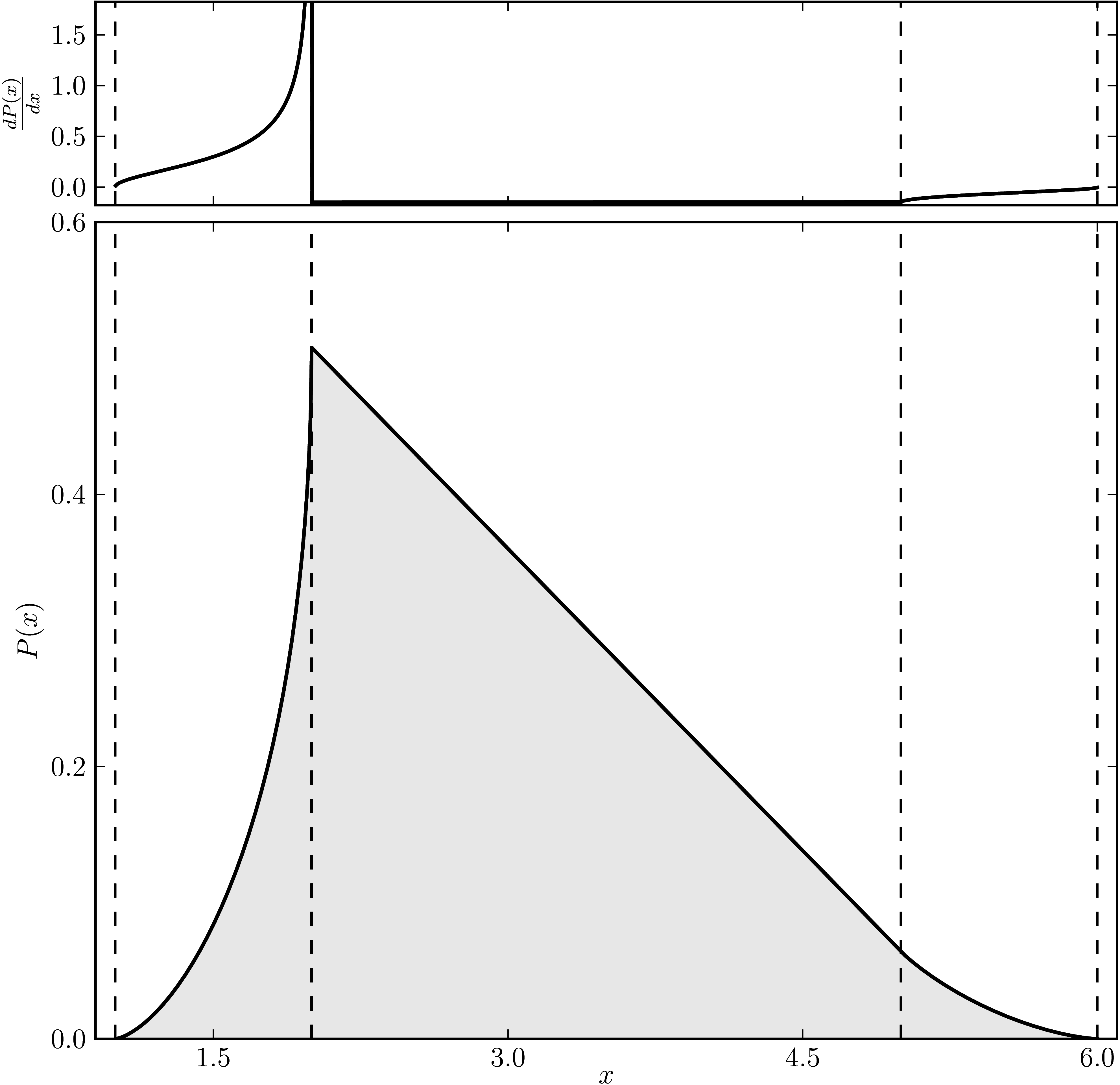### Example 5

The matrix is $\mathrm{diag}(1, 2, 2, 2 ,2, 6)$. The dashed lines mark the eigenvalues. The upped plot shows the derivative of the distribution.

Standard numerical shadow with respect to complex states Numerical shadow with respect to real states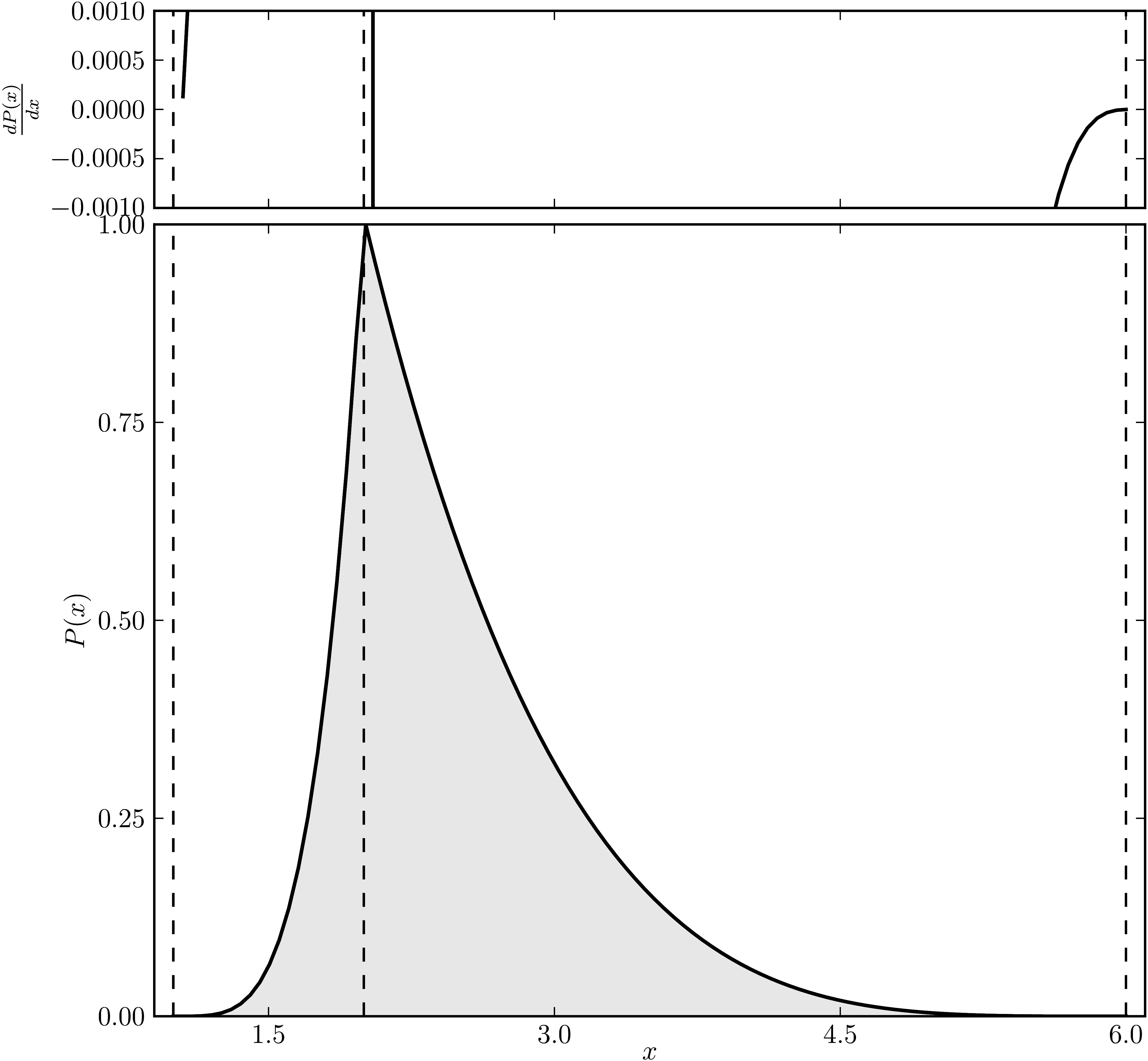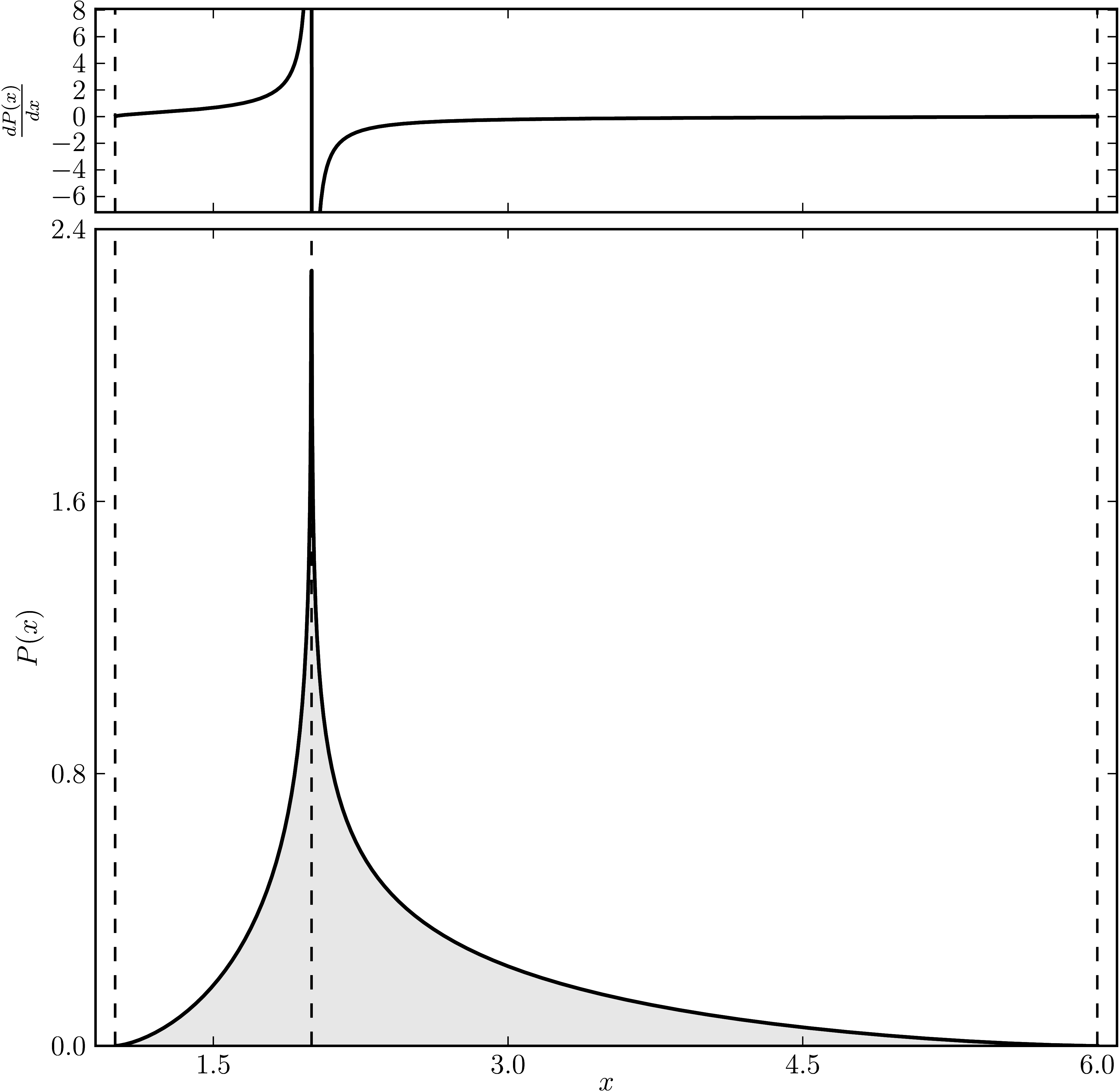### Example 6

The matrix is $\mathrm{diag}(2, 2, 2, 4 ,4, 4)$. The dashed lines mark the eigenvalues. The upped plot shows the derivative of the distribution.

Standard numerical shadow with respect to complex states Numerical shadow with respect to real states